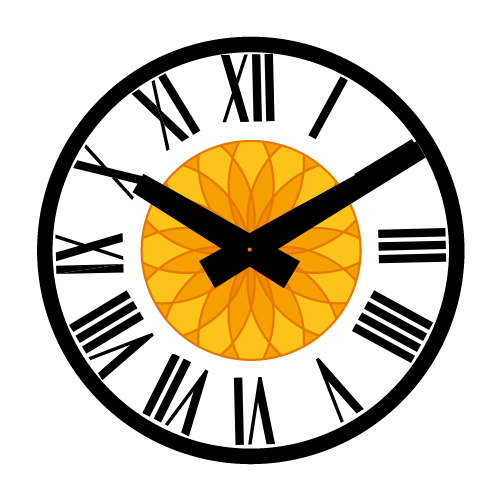Starting from this modelStarting from this modelStarting from this modelStarting from this modelStarting from this modelStarting from this modelStarting from this modelStarting from this modelStarting from this modelStarting from this modelStarting from this modelStarting from this model